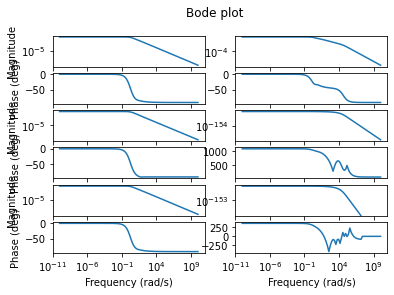/ pymor Public

Merged
merged 11 commits into from Aug 26, 2020
Merged

merged 11 commits into from Aug 26, 2020

## Conversation

This file contains bidirectional Unicode text that may be interpreted or compiled differently than what appears below. To review, open the file in an editor that reveals hidden Unicode characters. Learn more about bidirectional Unicode characters

###pmli commented Aug 7, 2020 • edited

This PR adds the `bode_plot` method to `InputOutputModel` for drawing the Bode plot (with both magnitude and phases).

Also, an example is added to the BT tutorial. I used the `constrained_layout` option since the suptitle is otherwise too high, but it is an experimental feature. The documentation suggests getting axes positions and setting them explicitly for reproducibility, but I guess this would have to be done for all 12 subplots...

See #389.

Update: This PR also adds the `bode` method that returns magnitudes and phases used in `bode_plot`.

# Codecov Report

Merging #1051 into master will decrease coverage by `0.16%`.
The diff coverage is `7.69%`.

Impacted Files Coverage Δ
src/pymor/models/iosys.py `45.36% <7.69%> (-2.14%)` ⬇️
src/pymor/vectorarrays/list.py `84.15% <0.00%> (-0.19%)` ⬇️
src/pymor/vectorarrays/numpy.py `84.55% <0.00%> (+0.24%)` ⬆️

### lbalicki commented Aug 22, 2020

 Overall the Bode plot seems to work well. Maybe we should change the default options of the plot a little bit, such that it looks better for more examples? With the default options of the current implementation, the example fom from the BT tutorial and `w = np.logspace(10, -10, 100)` in a Jupyter notebook I get the plot:I would suggest initializing the `fig, ax` objects via `fig, ax = plt.subplots(2 * self.output_dim, self.input_dim, sharex=True, figsize=(3 * self.input_dim, 4 * self.output_dim), constrained_layout=True)`, which for me results in "nice" looking graphs for a lot of different input and output dimensions. What do you think?

 fig = plt.gcf() ax = fig.subplots(2 * self.output_dim, self.input_dim, sharex=True, squeeze=False)

###lbalicki Aug 22, 2020

Change to `fig, ax = plt.subplots(2 * self.output_dim, self.input_dim, sharex=True, figsize=(3 * self.input_dim, 4 * self.output_dim), constrained_layout=True)`?

### pmli commented Aug 24, 2020

 I would suggest initializing the `fig, ax` objects via `fig, ax = plt.subplots(2 * self.output_dim, self.input_dim, sharex=True, figsize=(3 * self.input_dim, 4 * self.output_dim), constrained_layout=True)`, which for me results in "nice" looking graphs for a lot of different input and output dimensions. What do you think? Sounds good. Maybe it would be good to also use `plt.rcParams['figure.figsize']`. I'll try and see what works.

### pmli commented Aug 25, 2020

 Now the default Bode plot size is `[self.input_dim * width, 2 * self.output_dim * height]`, where `width, height = plt.rcParams['figure.figsize']` and `constrained_layout` is used. It seems that `constrained_layout` makes the subplots bigger, probably because it fills the margins. I'm not sure how to fix this besides just scaling the width and height. Additionally, I found that `np.unwrap` can make a sequence of phases more continuous and moved the computation of magnitudes and phases to a separate `bode` method (similar to `scipy.signal.bode`).

added 11 commits August 25, 2020 15:29
``` [models.iosys] add bode_plot method ```
``` 7f40453 ```
``` [docs] add Bode plot to BT tutorial ```
``` c2a9f5b ```
``` [docs] edit description of Bode plot ```
``` f524e76 ```
``` [docs] use constrained layout for Bode plot ```
``` 54e1697 ```
``` [models.iosys] use np.unwrap in bode_plot ```
``` 42466fb ```
``` [docs] turn grid on globally in BT tutorial ```
``` 3d1aafe ```
``` [models.iosys] add bode method ```
``` b0bc3bd ```
``` [models.iosys] remove unused imports ```
``` 6b37a9f ```
``` [models.iosys] edit bode_plot default figsize ```
``` 0e8761d ```
``` [docs] remove calling plt.subplots before bode_plot in BT tutorial ```
``` 9321ba1 ```
``` [docs] add more Bode plots in BT tutorial ```
``` e774b60 ```